# Python | Pandas Index.argsort()

• Last Updated : 29 Nov, 2019

Python is a great language for doing data analysis, primarily because of the fantastic ecosystem of data-centric python packages. Pandas is one of those packages and makes importing and analyzing data much easier.

Pandas` Index.argsort()` function returns the integer indices that would sort the index. By default the sorting order has been set to increasing order.

Syntax: Index.argsort(*args, **kwargs)

Parameters :
*args : Passed to numpy.ndarray.argsort
**kwargs : Passed to numpy.ndarray.argsort

Returns : numpy.ndarray
Integer indices that would sort the index if used as an indexer

Example #1: Use `Index.argsort()` function to find the order of indices which would sort the given Index.

 `# importing pandas as pd ` `import` `pandas as pd ` ` `  `# Creating the Index ` `df ``=` `pd.Index([``17``, ``69``, ``33``, ``5``, ``10``, ``74``, ``10``, ``5``]) ` ` `  `# Print the Index ` `df `

Output :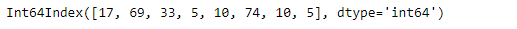Let’s find the ordering of the indices which would sort the Index.

 `# to find the ordering of indices  ` `# that would sort the df Index ` `df.argsort() `

Output :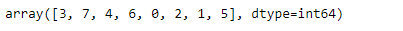As we can see in the output, the function has returned the ordering of the indices which would sort the given Index. We can verify that by printing the Index based on the ordering.

 `# Printing the Index based on the ` `# result of the argsort() function ` `df[df.argsort()] `

Output :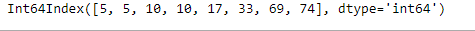As we can see in the output, it is printed in a sorted order.

Example #2: Use `Index.argsort()` function to find the order of indices which would sort the given Index.

 `# importing pandas as pd ` `import` `pandas as pd ` ` `  `# Creating the Index ` `df ``=` `pd.Index([``'Sam'``, ``'Alex'``, ``'Olivia'``, ` `          ``'Dan'``, ``'Brook'``, ``'Katherine'``]) ` ` `  `# Print the Index ` `df `

Output :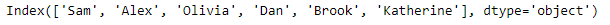Let’s find the ordering of the indices which would sort the Index.

 `# to find the ordering of indices ` `# that would sort the df Index ` `df.argsort() `

Output :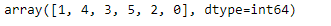As we can see in the output, the function has returned the ordering of the indices which would sort the given Index. We can verify that by printing the Index based on the ordering.

 `# Printing the Index based on the  ` `# result of the argsort() function ` `df[df.argsort()] `

Output :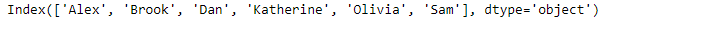As we can see in the output, it is printed in a sorted order.

My Personal Notes arrow_drop_up
Recommended Articles
Page :